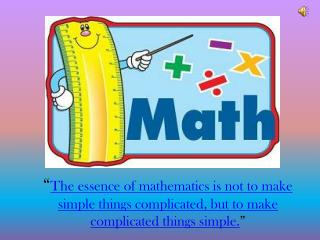Download Presentation“ The essence of mathematics is not to make simple things complicated, but to make complicated things simple. ”# “ The essence of mathematics is not to make simple things complicated, but to make complicated things simple. ” - PowerPoint PPT Presentation

Download Presentation##### “ The essence of mathematics is not to make simple things complicated, but to make complicated things simple. ”

Download Policy: Content on the Website is provided to you AS IS for your information and personal use and may not be sold / licensed / shared on other websites without getting consent from its author. While downloading, if for some reason you are not able to download a presentation, the publisher may have deleted the file from their server.

- - - - - - - - - - - - - - - - - - - - - - - - - - - E N D - - - - - - - - - - - - - - - - - - - - - - - - - - -
##### Presentation Transcript

1. “The essence of mathematics is not to make simple things complicated, but to make complicated things simple.”

2. DAMHS PHASE 4 Subject Specialist:mrs.FARIDAkhursheedSyndicate Leader:Mrs.UzmaAUsamaPresented by: Mrs. UzmaJalil

3. Topic: Rules Of Divisibility

4. OPERATIONAL FACTS On average, out of every 100 arithmetical problems you will encounter 70 require addition, 20 will require multiplication, 5 will require subtraction, and 5 will require division. Although least used, division is a part of everyday life. Parents, in providing inheritances for their children, generally seek to divide the estate equally between their children. When four people play with a deck of 52 cards, they seek to divide the cards equally to each player.

5. We are now ready to explore the nature of division and, to speak truthfully, division is one of the hardest processes for a student to master because it is the most complicated of all the arithmetical operation Since division is the inverse of multiplication, we can check a division problem using multiplication . A number is said to be divisible by another number when, after dividing, the remainder is zero Divisibility is a key property of whole numbers. Understanding divisibility will help you master division. Here a question arises in mind that…….. what is divisibility?

6. What does divisibility mean? It means that after dividing, there will beNOremainder.

7. How divisibility rules are useful! Divisibility rules of whole numbers are very useful because they help us to quickly determine if a number can be divided by: 2,3,4,5,6,8,9,10,12,15….. Without doing long division no matter how long the number is to understand this we have to learn the rules of divisibility, so we will first start with the 1st Rule of divisibility with 2 .

8. Rule of divisibility by 2 • A number is divisible by 2 if it's last digit is an EVEN • number. • Even numbers are 0,2,4,6,8…………………….. The best • example of even numbers is table of 2. 2x1=02 2x2=04 2x3=06 2x4=08 2x5=10 2x6=12 2x7=14 2x8=16 2x9=18 2x10=20

9. Rules Of Divisibility By 5 & 10 • A number is divisible by 5 if its last digit is “ 0 or 5” • For instance, 9564655 is divisible by “5” because • the last digit is “5” or 2345670 is also divisible by • “5” because its last digit is “0”. • A number is divisible by 10 if its last digits is “0”.For instance, 522480 is divisible by 10 because the last digit is 0.

10. ACTIVITY TIME

11. Rules Of Divisibility By 3 & 9 A number is divisible by 3 if the sum of its digits is divisible by 3 or their sum is 3,6 or 9.For instance, 3141 is divisible by 3 because 3+1+4+1 = 9 and 9 is divisible by 3. A number is divisible by 9 if the sum of its digits is divisible by 9 or in other words we can say if the answer is 9.For instance, 20880 is divisible by 9 because the sum of its digits is divisible by 9 2+0+8+8+0=18 or (1+8=9). 817020 8+1+7+0+2+0= 18 3 & 9 653142 6+5+3+1+4+2= 21 3 only 840213 8+4+0+2+1+3=18 3 & 9 115307 1+1+5+3+0+7=17 None

12. Rules Of Divisibility By 6,12 & 15 • A number is divisible by 6 if it is divisible by 2 and 3 not only one of them. Eg 12 it is divisible by 2 &3 both therefore it is divisible by 6 also, but 4 is divisible by 2, not 3 therefore it is not divisible by 6 same is the case with 9 it is divisible by 3 not 2 therefore it is not divisible by 6. • If the number is divisible by 3 and by 4 both than it is divisible by 12 also. Eg 24 it is divisible by 3 & 4 both therefore it is divisible by 12 also , but 16 is divisible by only 4 not 3 so it is not divisible by 12. • If the number is divisible by 3 and by 5 both than it is divisible by 15 also. Eg 30 it is divisible by 3 & 5 both therefore it is divisible by 15 also , but 12 is divisible by only 3 not 5 so it is not divisible by 15.

13. Rules Of Divisibility By 4 & 8 • A number is divisible by 4 if the number represented by its last two digits is divisible by 4.For instance, 8920 is divisible by 4 because 20 is divisible by 4. • A number is divisible by 8 if the number represented by its last three digits is divisible by 8. For instance, 587320 is divisible by 8 because 320 is divisible by 8.

14. ACTIVITY TIME

15. Reference Sites http://www.biblicalchristianworldview.net/Mathematical-Circles/divisibilityRules.pdf en.wikipedia.org/wiki/Divisibility_rule - Cached - Similar http://www.helpingwithmath.com/by_subject/division/div_divisibility_rules.htm